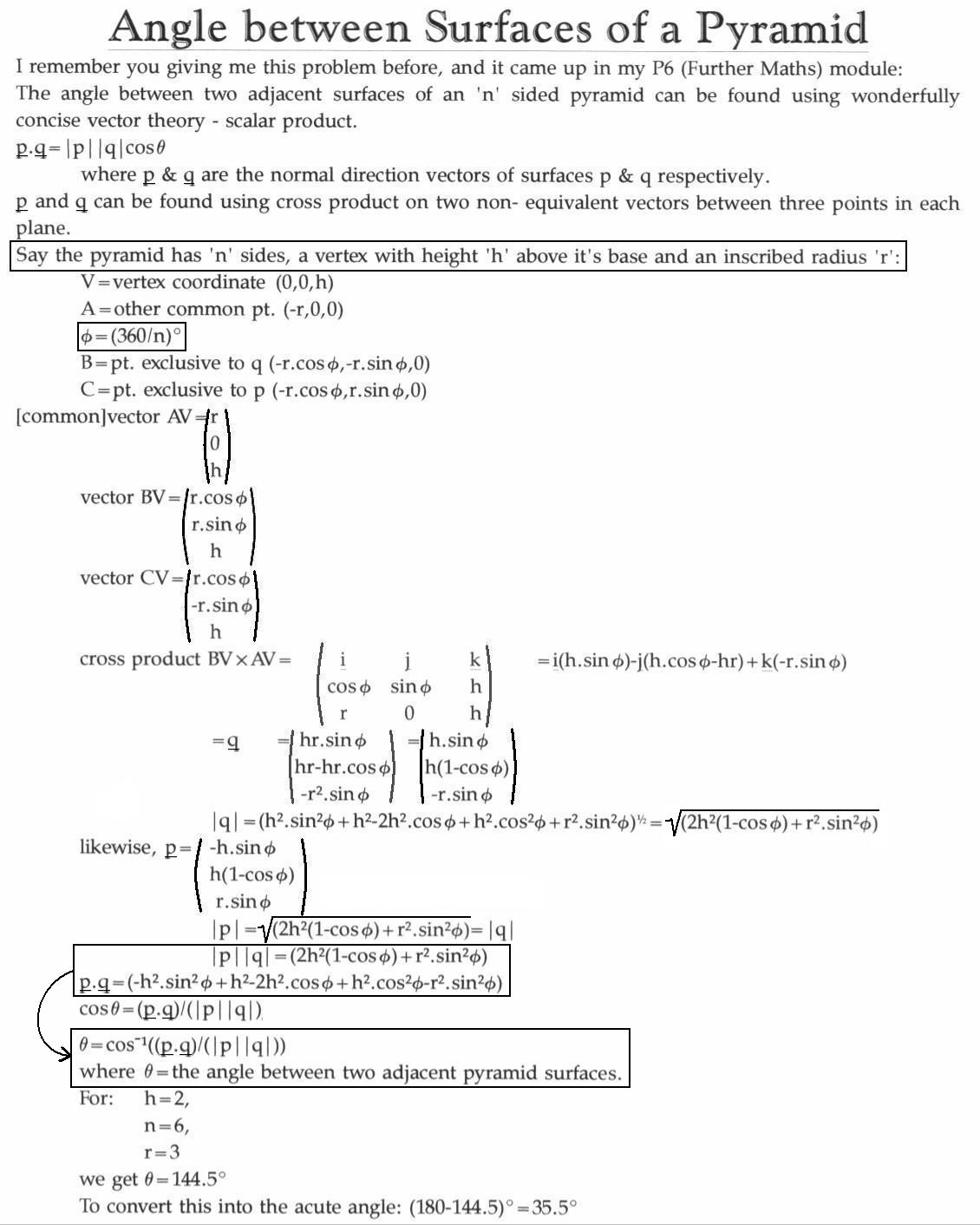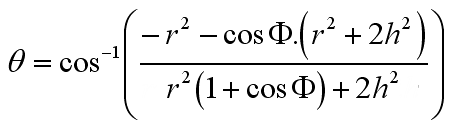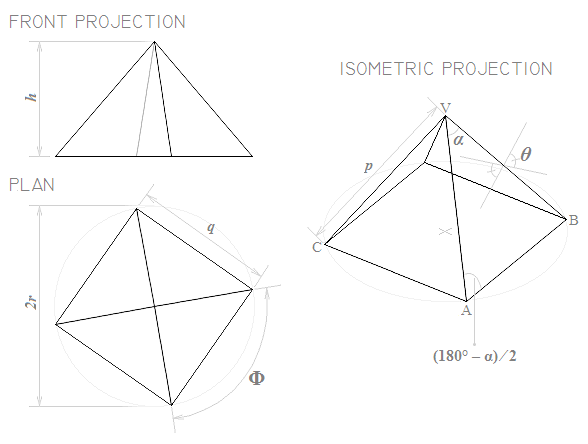Otherwise called “compound saw angles”

A cabinet-maker wanted to find the angle to chamfer the edges of two or more adjoining pieces of wood to make them neatly fit together, forming a regular pyramid.
My High School maths teacher Mr. Stephen Bell posed this challenge to me in 1994, from his colleague (Mr Kennedy the craft teacher), who had been unable to solve the problem analytically.  I was unable to solve the problem using classical geometry / trigonometry at age sixteen, but two years later, while studying for the A-Level Further Maths qualification, we were equipped with the tools necessary to answer this question (a combination of vector products: scalar product and cross product).  First, the cross-product is used to obtain surface-normal vectors for adjacent upward-facing surfaces of the pyramid, and then these normal vectors are compared using scalar-product to determine the angle between the surfaces.
These workings are accurate for regular pyramids (i.e. those comprising similar equilateral or isosceles triangles atop a polygon-shaped base).  A similar method might be applied to general compound saw angle type problems.Therefore (applying a trigonometric identity):Applying a further trigonometric identity, a difference of two squares and a factorisation or two:As r tends towards zero, Theta tends towards Phi.  However, where r is not equal to zero, we may safely apply a further factorisation to express Theta in terms of cos(Phi) and (h/r)2: (by dividing the top and bottom by r2):Angle Theta
The smallest angle between upper faces of the pyramid, internal to the pyramid (i.e. in a plane perpendicular to the surfaces in question).
Angle Phi
360° divided by n (the number of sides on the regular pyramid).
n.b. 2pi is a mathematical way of writing 360°, expressing this angle in radians
h = Height of pyramid (perpendicular to the base)
The smallest distance from the base of the pyramid, to the vertex which does not form part of the base (i.e. measured at right angles to the base).
r = Inscribed radius of pyramid
The distance from the centre of the base of the pyramid, to one of the vertices on the base = the radius of the smallest circle into which the (regular polygon) base of the pyramid will fit.
n = Number of sides
Total number of faces, minus one (the base).
cos-1
Standard mathematical shorthand for (Inverse cosine) of the angle that follows

The chamfer angle (for the solution to the cabinet maker's problem) may be found by dividing by angle Theta by two (since two surfaces meet at that point).Now using “p” and “q” to mean something different to what they meant before:The inscribed radius and perpendicular height of an n-sided pyramid may be found using the following formulae:p
The length of each upper (sloping) edge (corner) of the pyramid (or each edge of the polyhedron)
q
The length of each side of the pyramid's base (or, for a polyhedron, the distance between a vertex and the next-but-one vertex around a face that contains that vertex)
Alpha
The uppermost internal angle of each triangle comprising an upper face of the pyramid (e.g. 60° in the case of an equilateral triangle i.e. p=q; or 90° for the corners of a cube.)

Therefore Theta may be expressed purely in terms of

• Phi and Alpha;
• or in terms of n, h and r;
• or more conveniently for the exercises that follow, in terms of n (the number of sides) and Alpha (the uppermost vertex angle on each triangle) of the pyramid.

Pyramid dimensioning calculator:

Caveats: Distance measurements are in arbitrary units (calculations are valid regardless of the units, provided that all the measurements are supplied in the same units of distance measurement.  The results will be in these same units.)  In other words, you may calculate according to a unitary-sized pyramid and scale the distance-measurements accordingly.  (Remember to scale area factor according to the square of the scale factor, and volume or mass according to the cube of the scale factor.)
The results are rounded to 8 significant figures by default, so as to avoid misleading you into thinking these calculations are internally more precise than they really are.  If you want higher precision for feeding into further calculation steps, you should perform the calculations manually...  (Anyone competent to use greater precision should be competent to do so.)
Ideally, this calculator should accept any defining set of dimensions and tell you the dimensions you didn't specify - this is planned for the next round of updates.

If you enter all input measurements, the calculator will produce an scaled isometric projection of your pyramid, so that you can verify (approximately) that the measurements are as you intended.

 > - Required Field > Angle Alpha atapex of upper faces: ° > No. of sideson base polygon n: Upper edgemeasurement p: Angle Theta: Chamfer Angle: Perp. height h: Inscribed radius r: Distance q:"I have not failed, I have only found 10,000 ways that do not work." - Thomas A. Edison This website works best with JavaScript enabled.  © 2004-2019 Matthew Slyman